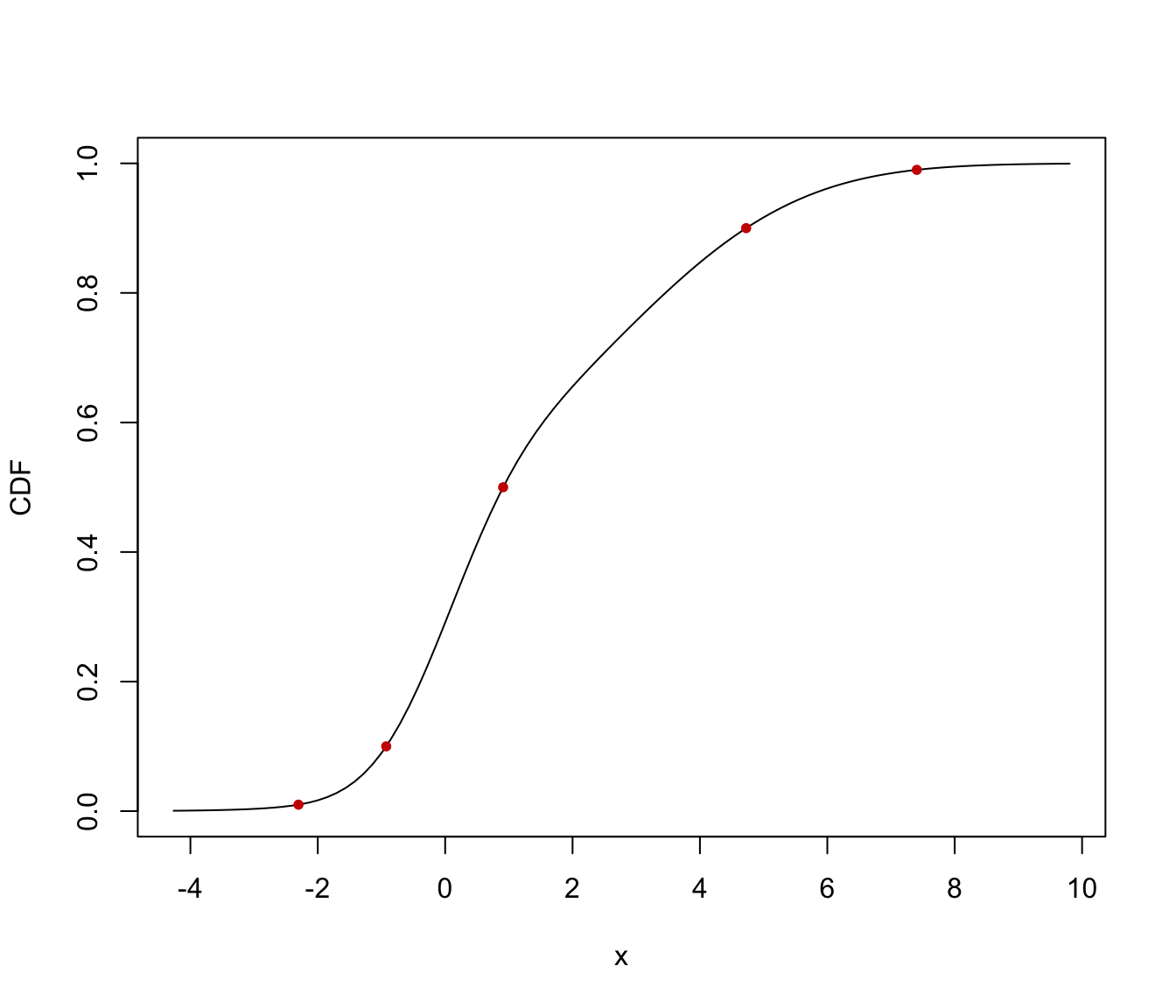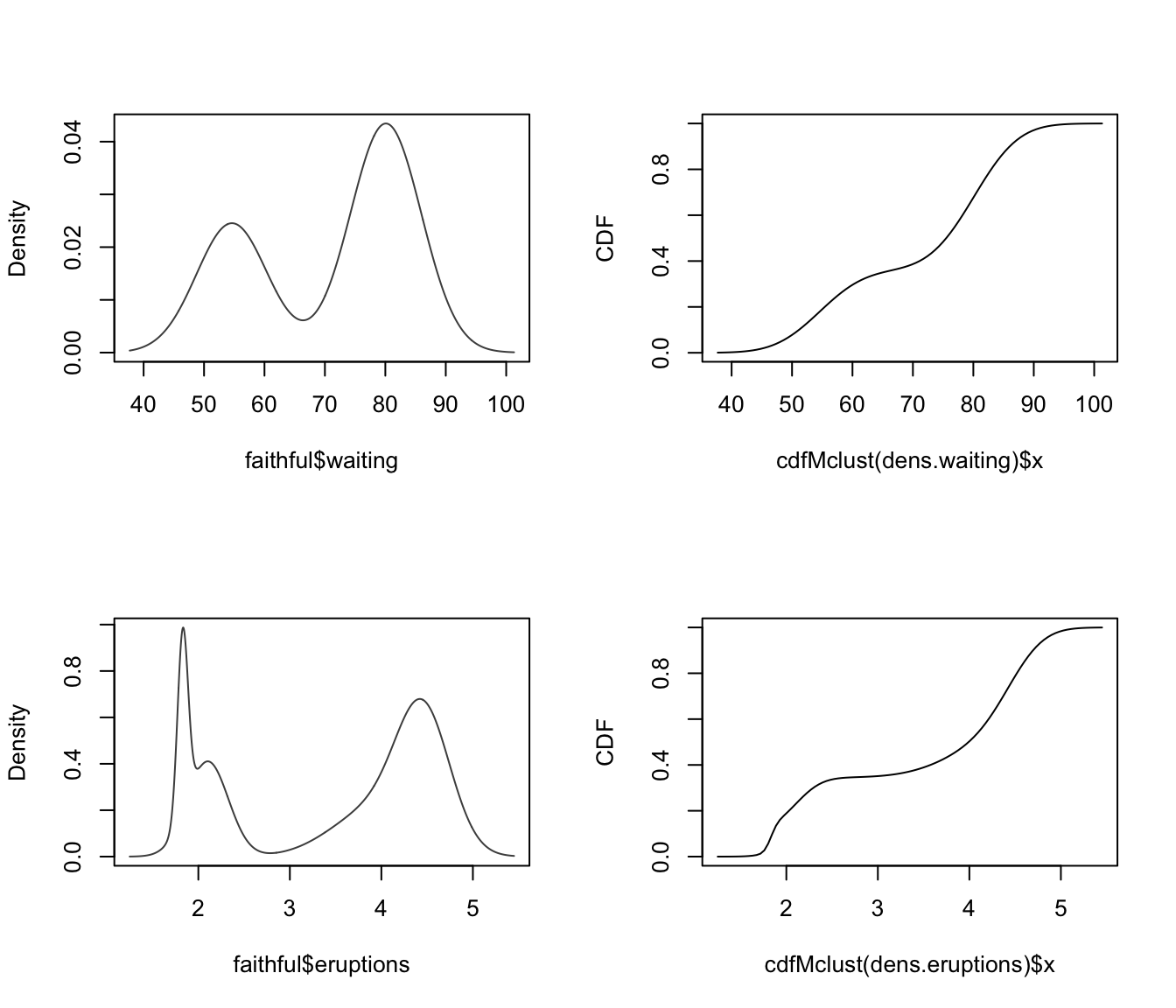Compute the cumulative density function (cdf) or quantiles from an estimated one-dimensional Gaussian mixture fitted using densityMclust.

cdfMclust(object, data, ngrid = 100, ...)
quantileMclust(object, p, ...)

Arguments

object

a densityMclust model object.

data

a numeric vector of evaluation points.

ngrid

the number of points in a regular grid to be used as evaluation points if no data are provided.

p

a numeric vector of probabilities.

...

further arguments passed to or from other methods.

Details

The cdf is evaluated at points given by the optional argument data. If not provided, a regular grid of length ngrid for the evaluation points is used.

The quantiles are computed using bisection linear search algorithm.

Value

cdfMclust returns a list of x and y values providing, respectively, the evaluation points and the estimated cdf. quantileMclust returns a vector of quantiles.

Luca Scrucca

Examples

x <- c(rnorm(100), rnorm(100, 3, 2))
dens <- densityMclust(x)summary(dens, parameters = TRUE)
#> -------------------------------------------------------
#> Density estimation via Gaussian finite mixture modeling
#> -------------------------------------------------------
#>
#> Mclust V (univariate, unequal variance) model with 2 components:
#>
#>  log-likelihood   n df       BIC      ICL
#>       -424.0276 200  5 -874.5469 -942.139
#>
#> Mixing probabilities:
#>         1         2
#> 0.4813092 0.5186908
#>
#> Means:
#>          1          2
#> -0.0617169  2.8923878
#>
#> Variances:
#>         1         2
#> 0.8938594 4.3252118
cdf <- cdfMclust(dens)
str(cdf)
#> List of 2
#>  $x: num [1:100] -3.8 -3.66 -3.52 -3.38 -3.25 ... #>$ y: num [1:100] 0.000357 0.000458 0.000591 0.000766 0.001 ...
q <- quantileMclust(dens, p = c(0.01, 0.1, 0.5, 0.9, 0.99))
cbind(quantile = q, cdf = cdfMclust(dens, q)$y) #> quantile cdf #> [1,] -2.1730968 0.01 #> [2,] -0.9526056 0.10 #> [3,] 0.9295521 0.50 #> [4,] 4.6968529 0.90 #> [5,] 7.1950392 0.99 plot(cdf, type = "l", xlab = "x", ylab = "CDF") points(q, cdfMclust(dens, q)$y, pch = 20, col = "red3")par(mfrow = c(2,2))
dens.waiting <- densityMclust(faithful$waiting) plot(dens.waiting)plot(cdfMclust(dens.waiting), type = "l", xlab = dens.waiting$varname, ylab = "CDF")
dens.eruptions <- densityMclust(faithful$eruptions) plot(dens.eruptions)plot(cdfMclust(dens.eruptions), type = "l", xlab = dens.eruptions$varname, ylab = "CDF")par(mfrow = c(1,1))